# Extra Questions for Class 6 Chapter 4 Mathematics## myCBSEguide

Trusted by 70 Lakh Students

Extra Questions for Class 6 Chapter 4 Mathematics. myCBSEguide has just released Chapter Wise Question Answers for class 6 Maths. There chapter wise Practice Questions with complete solutions are available for download in myCBSEguide website and mobile app. These Extra Questions with solution are prepared by our team of expert teachers who are teaching grade in CBSE schools for years. There are around 4-5 set of solved Mathematics Extra questions from each and every chapter. The students will not miss any concept in these Chapter wise question that are specially designed to tackle Exam. We have taken care of every single concept given in CBSE Class 6 Mathematics syllabus and questions are framed as per the latest marking scheme and blue print issued by CBSE for Class 6.

CBSE Class 6 Maths Extra Questions

## Basic Geometrical Ideas Class 6 Maths Important Questions

Basic Geometrical Ideas

1. Which of the pair of adjacent angles in the given figure?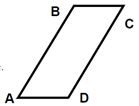1. {tex}\angle {/tex}B, {tex}\angle {/tex}D
2. {tex}\angle {/tex}B, {tex}\angle {/tex}C
3. None of these
4. {tex}\angle {/tex}A, {tex}\angle {/tex}C
2. How many lines pass through one given point?
1. Two
2. One
3. Three
4. Count less
3. Point P is _____________.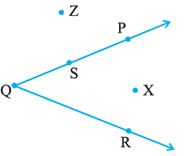1. in the exterior of the angle
2. on the angle
3. in the interior of the angle
4. away from the angle
4. Measure of the two angles between hour and minute hands of a clock at 9 O’ clock are
1. {tex}270 ^ { \circ } , 90 ^ { \circ }{/tex}
2. {tex}60 ^ { \circ } , 300 ^ { \circ }{/tex}
3. {tex}75 ^ { \circ } , 285 ^ { \circ }{/tex}
4. {tex}30 ^ { \circ } , 330 ^ { \circ }{/tex}
5. Radius of a circle is ________.
1. half its diameter
2. thrice its diameter
3. one-fourth its diameter
4. 4 times its diameter.
6. Match the following:-

Column AColumn B
1. Every circle has a point at(a) Diameter
2. Line segment passing through the centre of a circle(b) Centre
3. Half of the diameter(c) Arc
4. The path in the circle formed from two points on the circle(d) Radius
7. Fill up the following:

1. ________ has no length, breadth, height or thickness.
2. A line segment has a definite ___________ .
3. Curves that do not intersect themselves are called ___________ curves.
4. An ‘angle’ is made up of ___________ rays having a common end point.
8. State true or false:

1. A point indicates a definite position.
2. A line segment is a part of a plane.
3. A line is a set of points closely arranged.
4. Two lines in a plane always intersect in a point.
9. Classify the following curves as

1. open
2. closed.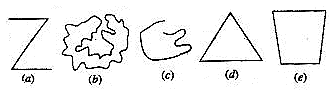10. Draw rough diagrams to illustrate the following :

1. Open curve.
2. Closed curve.
11. How many end points a line segment have?
12. Illustrate, if possible, each one of the following with a rough diagram :

1. A closed curve that is not a polygon.
2. An open curve made up entirely of line segments.
3. A polygon with two sides.
13. From the figure identify

1. the centre of circle.
3. a diameter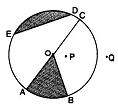14. Write the points which are: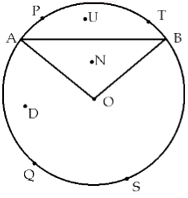1. in the minor sector OAPB
2. minor segment ATB
3. major sector OAQB
4. major arc AQB
5. minor arc APB
15. Define the following terms:

1. Line segment,
2. Line,
3. Intersecting lines,
4. Parallel lines

Basic Geometrical Ideas

1. {tex}\angle {/tex}B, {tex}\angle {/tex}C
Explanation: Adjacent angles have a common side. In this figure side BC contain two angles which are angle B and angle C making them adjacent angles.
1. Count less
Explanation: through a sinlge point infinite number of lines can be passed by Euclid’s axiom.
1. on the angle
Explanation: point P lies on the ray QP of the angle therefore P is on the angle
1. (a) {tex}270 ^ { \circ } , 90 ^ { \circ }{/tex}
Explanation:{tex}270 ^ { \circ } , 90 ^ { \circ }{/tex}
1. half its diameter
Explanation: Radius is from the center of the circle to the circle’s edge. The diameter is edge to edge with the line going through the radius. Diameter is twice the length of the radius or 2r where r is the radius of the circle.
1. {tex} \to {/tex} (b)
2. {tex} \to {/tex} (a)
3. {tex} \to {/tex} (d)
4. {tex} \to {/tex} (c)
1. Point
2. length
3. simple
4. two
1. True
2. False. A line segment is a part of a line that has two end points
3. False. A line is a straight path that is endless
4. False Two lines in a plane intersect in a point or parallel.
1. a, c
2. b, d, e
1.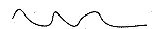2.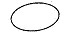1. 2
1.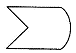2.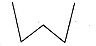3. Not possible.
1. O is the centre of the circle.
2. {tex}\overline{\mathrm{OA}}, \overline{\mathrm{OB}}, \overline{\mathrm{OC}}{/tex} are three radii of the circle.
3. {tex}\overline{A C}{/tex} is the diameter of the circle.
1. U and N
2. U
3. D
4. A, Q, S and B
5. A, P, T and B
1. Line segment: A straight line drawn from any point to any other point is called as line segment.
2. Line: Line is a straight path of points that goes on forever in two directions. It has infinite length, but no breadth and height.
3. Intersecting lines: Interesting lines are lines that pass through the same point.
4. Parallel lines: Parallel lines are never cross and always stay the same distance apart.

## Chapter Wise Extra Questions for Class 6 Mathematics## Test Generator

Create Tests with your Name & Logo## Job Openings at myCBSEguide

We are hiring Subject Matter Experts and Video Content Creators for our Dwarka, New Delhi office.